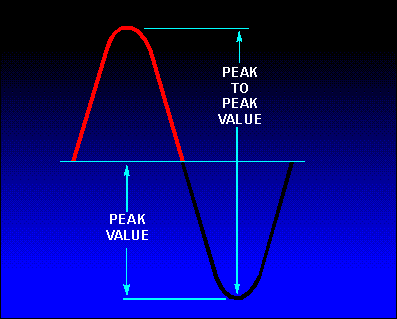Alternating Current ValuesCustom SearchALTERNATING CURRENT VALUES In discussing alternating current and voltage, you will often find it necessary to express the current and voltage in terms of MAXIMUM or PEAK values, PEAK-to-PEAK values, EFFECTIVE values, AVERAGE values, or INSTANTANEOUS values. Each of these values has a different meaning and is used to describe a different amount of current or voltage. PEAK AND PEAK-TO-PEAK VALUES Refer to figure 1-13. Notice it shows the positive alternation of a sine wave (a half-cycle of ac) and a dc waveform that occur simultaneously. Note that the dc starts and stops at the same moment as does the positive alternation, and that both waveforms rise to the same maximum value. However, the dc values are greater than the corresponding ac values at all points except the point at which the positive alternation passes through its maximum value. At this point the dc and ac values are equal. This point on the sine wave is referred to as the maximum or peak value. Figure 1-13. - Maximum or peak value.During each complete cycle of ac there are always two maximum or peak values, one for the positive half-cycle and the other for the negative half-cycle. The difference between the peak positive value and the peak negative value is called the peak-to-peak value of the sine wave. This value is twice the maximum or peak value of the sine wave and is sometimes used for measurement of ac voltages. Note the difference between peak and peak-to-peak values in figure 1-14. Usually alternating voltage and current are expressed in EFFECTIVE VALUES (a term you will study later) rather than in peak-to-peak values. Figure 1-14. - Peak and peak-to-peak values.Q.24 What is meant by peak and peak-to-peak values of ac? Q.25 How many times is the maximum or peak value of emf or current reached during one cycle of ac? INSTANTANEOUS VALUE The INSTANTANEOUS value of an alternating voltage or current is the value of voltage or current at one particular instant. The value may be zero if the particular instant is the time in the cycle at which the polarity of the voltage is changing. It may also be the same as the peak value, if the selected instant is the time in the cycle at which the voltage or current stops increasing and starts decreasing. There are actually an infinite number of instantaneous values between zero and the peak value. AVERAGE VALUE The AVERAGE value of an alternating current or voltage is the average of ALL the INSTANTANEOUS values during ONE alternation. Since the voltage increases from zero to peak value and decreases back to zero during one alternation, the average value must be some value between those two limits. You could determine the average value by adding together a series of instantaneous values of the alternation (between 0° and 180°), and then dividing the sum by the number of instantaneous values used. The computation would show that one alternation of a sine wave has an average value equal to 0.636 times the peak value. The formula for a verage voltage is Eavg = 0.636 X Emax where Eavg is the average voltage of one alternation, and Emax is the maximum or peak voltage. Similarly, the formula for average current is Iavg = 0.636 X Imax where Iavg is the average current in one alternation, and Imax is the maximum or peak current. Do not confuse the above definition of an average value with that of the average value of a complete cycle. Because the voltage is positive during one alternation and negative during the other alternation, the average value of the voltage values occurring during the complete cycle is zero. Q.26 If any point on a sine wave is selected at random and the value of the current or voltage is measured at that one particular moment, what value is being measured? Q.27 What value of current or voltage is computed by averaging all of the instantaneous values during the negative alternation of a sine wave? Q.28 What is the average value of all of the instantaneous currents or voltages occurring during one complete cycle of a sine wave? Q.29 What mathematical formulas are used to find the average value of current and average value of voltage of a sine wave? Q.30 If Emax is 115 volts, what is Eavg? Q.31 If Iavg is 1.272 ampere, what is Imax ?Integrated Publishing, Inc.